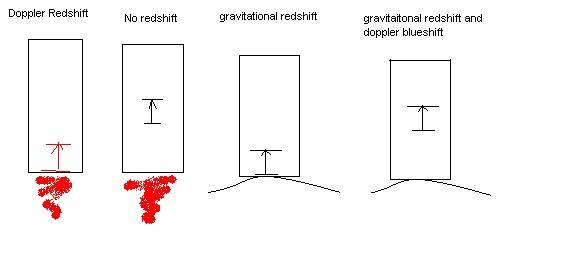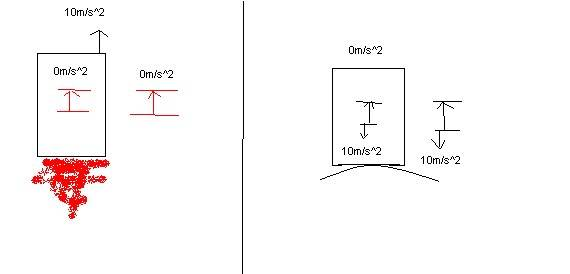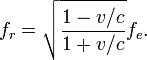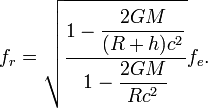# Redshift and Weak equivalence principle

Firstly: Does anyone know at our current level of technology what "small enough not to detect tidal forces" equates to? Near the surface of the earth(as it probably depends on the amount of curvature of space).

Secondly: Does anyone know at out current level of technology what the minimum change in height required for a measurable difference in redshift? Also near the surface of the earth as it depends on amount of curvature.

A way to tell between gravity and a pseudo-force(aside from tidal effects):

Depending on how short a distance we can detect redshift from gravity one could make a self-contained device I'll call it a Redshiftometer that sends a photon of known wave-length and measures it some distance on the other side of the device, all internally.

In the case of a rocket either accelerating or on the surface of the earth if you were to climb to the top of your rocket and let go, and then there is a time that you are either falling to the bottom of the rocket, or the rocket's bottom is accelerating toward you. During this time you can compare the readings on an Accelerometer and the Redshiftometer, in the case of an accelerating rocket it flat space, while in the air, experiencing what appears to be downward acceleration the Accelerometer reads no acceleration, and the Redshiftometer confirms it, however in the case of the rocket on earth the Accelerometer would read 0 while the Redshiftometer would indicate that gravity was present.

This should work for any other psuedo-force as well...

If that is the case, can we put the idea of gravity as a pseudo-force away, or does it prove nothing, or do we just add, "as long as you can't tell a redshift difference too" if thats what we do then it is not surprising that the statement "You can't tell between non-inertial frame motion and gravity so long as you constain the experiments such as that you can't tell the effects of gravity vesus a non-inertial frame" doesn't really surprise me and should not be the basis of any theory....

Matterwave
Gold Member
The light would be redshifted (or blue shifted depending on your set up) in the accelerating rocket....why wouldn't it redshift?

There are some tidal force figures for reference here:
http://en.wikipedia.org/wiki/Tidal_forces#Mathematical_treatment

but they don't explain experimental accuracy limits.

minimum change in height required for a measurable difference in redshift?

I think you mean minimum change in gravitational potential. And I'd guess you mean outside a black hole so it could be observed.....In any case, this can be related to differences in time so it's got to be, really,really small....

and Wiki sez this:
....Atomic clocks are the most accurate time and frequency standards known,......National standards agencies maintain an accuracy of 10−9 seconds per day (approximately 1 part in 1014), and a precision set by the radio transmitter pumping the maser.
http://en.wikipedia.org/wiki/Cesium_clock

Does anyone know if these standards a adjusted for differences in altitute here on earth?? [I did not read the whole Wiki article referenced.]

PeterDonis
Mentor
Secondly: Does anyone know at out current level of technology what the minimum change in height required for a measurable difference in redshift?

The Pound-Rebka experiment detected gravitational redshift over a height change of 22.5 meters in 1960:

http://en.wikipedia.org/wiki/Pound–Rebka_experiment

I would expect that today's equipment could detect it over a considerably smaller height change, but I haven't been able to find any specific experimental results.

A way to tell between gravity and a pseudo-force (aside from tidal effects):

Depending on how short a distance we can detect redshift from gravity one could make a self-contained device I'll call it a Redshiftometer that sends a photon of known wave-length and measures it some distance on the other side of the device, all internally.

In the case of a rocket either accelerating or on the surface of the earth if you were to climb to the top of your rocket and let go, and then there is a time that you are either falling to the bottom of the rocket, or the rocket's bottom is accelerating toward you. During this time you can compare the readings on an Accelerometer and the Redshiftometer, in the case of an accelerating rocket it flat space, while in the air, experiencing what appears to be downward acceleration the Accelerometer reads no acceleration, and the Redshiftometer confirms it, however in the case of the rocket on earth the Accelerometer would read 0 while the Redshiftometer would indicate that gravity was present.

If the redshiftometer is falling along with you, it will register zero redshift in both cases. You would have to leave the redshiftometer at rest relative to the rocket (i.e., not freely falling) in order to measure redshift in the "gravity" case, but if the redshiftometer is at rest relative to the rocket it will also measure a redshift in the "accelerating" case. So this scheme won't distinguish between "acceleration" and "gravity".

Last edited:
Alright, I drew some picutes and did some math:

The pound-rebka experiment worked with 25.5m of height, I did the math using 30m, with a more precise calculator and given precise measuring equipment the height could be loweredThis picture shows the four states that we are concerned with:

in 1 the device is being accelerated with the rocket, at rest with the system, and so doppler redshift occurs as the second plate has a velocity relative to the first plate(when the photon was fired) it is very negligible at 10m/s

in 2, the device is in "free-fall" compared to the accelerating system, at this point it is equivalent to being in flat-space, so no relative velocity, no redshift.

in 3 the object is at rest with the system, but gravitavitational redshift occurs, Even just comparing frames one and three one could tell the difference, the gravitational redshift resultant from an equal acceleration is greater than that from doppler.

in 4, we should expect the same results as in 2, but instead we see gravitational redshift, and a very slight doppler blueshift(i think) giving a non-zero result.This picture is to emphasize that while not physically touching any sides of the rocket the device acts as though it were in free space(in the first example), or accelerating toward earth in the second. The presence of doppler blueshift in the second example proves acceleration(right?)

Now for the math, I don't know how to use latex so it will be ugly...:

for doppler redshift:for gravitational:a = 10m/s^2
s = 30m
ds = s/c = 1e-7m
t = sqrt(2ds/a) = 1.41e-4s

v = at = 1.41e-3 m/s

M = 5.9e24Kg
G = 6.67e-11
MG = 3.94e14
R = 6.4e6m
h = 30m

Plug all this in you you get that v/c is really close to 0(4.7e-12), so the doppler redshift is nearly negligible, plugging in the numbers for gravitational, I wind up with fr = .966fe

This all comes down to:
Relativistic particles and photons do not fall like local masses. Photons in a gravitational field experience twice the local gravitational acceleration (tracing a null geodesic) - an important test of general relativity versus Newton. - Wikipedia on equivalence principle

So measuring the acceleration of photons(in the form of red/blueshift is the key to determining whether gravity is afoot...

I bring up other psuedoforces becuase in the case of centrifugal force, one would measure acceleration away from the center(a tidal force too, as it depends on r?) but, there should be no redshift from sending a photon from the edge of the circle to the center.

If the redshiftometer is falling along with you, it will register zero redshift in both cases.

Are you sure? That is what I originally feared, but I can't see how that would be the case, the doppler blueshift should not cancel out the gravitational redshift..

PAllen
Uniform acceleration by rocket affect produces exactly the same redshift/blueshift as a uniform gravitational field. A clock at the back of a rocket will run slower than a clock at the top. Of course, any experiment 'big enough' can distinguish uniform acceleration from non-uniform gravity, but that is not considered an issue with the equivalence principle.

The latest generation of super-accurate clocks can distinguish change in time flow over a height of 6 feet or less.

Uniform acceleration by rocket affect produces exactly the same redshift/blueshift as a uniform gravitational field. A clock at the back of a rocket will run slower than a clock at the top

I could not agree more given that the clocks were attached to the sides of the rocket, however, a rocket speeding past a clock in flat space(whether the clock is beside or inside does't matter as long as there is no contact(assuming no air or other means of contact) would not change it's rate(relative to itself)

PeterDonis
Mentor
Are you sure? That is what I originally feared, but I can't see how that would be the case, the doppler blueshift should not cancel out the gravitational redshift..

In general they won't, but under conditions where tidal gravity is unmeasurable, the difference between the two will also be unmeasurable. Your numerical calculations don't seem to show that, but I'm not sure those calculations are correct. Let me run through a derivation of formulas for both shifts. I'll work in units where G = c = 1, which makes things a lot easier to write.

The gravitational shift formula is:

$$G = \sqrt{\frac{1 - \frac{2M}{R + h}}{1 - \frac{2M}{R}}}$$

The Doppler shift formula is:

$$D = \sqrt{\frac{1 + v}{1 - v}}$$

Note that I have written both formulas so that the "shift" is greater than 1, so they can be directly compared. M is the mass of the Earth, R is the radius of the Earth, h is the change in height of the light ray during the measurement, and v is the change in speed of the detector during the measurement.

First of all, note that in units where c = 1, we have $v = ah$, where a is the acceleration due to gravity, because h is also the light travel time, and therefore the time during which the acceleration a changes the speed of the detector. Also, in units where G = 1, we have

$$a = \frac{M}{R^{2}}$$

so

$$v = \frac{M}{R^{2}} h$$

Second, note that the following relationships hold among the quantities in the formulas above: M << R, h << R, v << 1. That means we can expand out the formulas above by expanding out the square roots and dropping terms of second and higher order in small quantities, as follows:

$$G = \left( 1 - \frac{2M}{R + h} \right)^{\frac{1}{2}} \left( 1 - \frac{2M}{R} \right)^{- \frac{1}{2}} = \left( 1 - \frac{M}{R + h} \right) \left( 1 + \frac{M}{R} \right) = 1 - \frac{M}{R + h} + \frac{M}{R} = 1 + \frac{M}{R \left( R + h \right)} h = 1 + \frac{M}{R^{2}} h$$

$$D = \left( 1 + v \right)^{\frac{1}{2}} \left( 1 - v \right)^{- \frac{1}{2}} = \left( 1 + \frac{1}{2} v \right) \left( 1 + \frac{1}{2} v \right) = 1 + v = 1 + \frac{M}{R^{2}} h$$

So to the order of approximation given, the two shifts are the same. Errors in G will be of order M/R or h/R, and errors in D will be of order v, which is the product of M/R and h/R. The key, though, is that tidal effects go like Mh / R^3 for objects separated by distance h; in other words, tidal effects are of size h / R compared to the redshift, and h/R is the largest of the error terms (since h is larger than M--for Earth, M is of order 10^-2 meters, and h is 30 meters). So, as I said at the start of this post, if tidal effects are negligible, the variation in gravitational redshift that would make it detectable vs. Doppler blueshift in your scenario are also negligible.

Last edited:
That is approximation just shows that it is negligible in small enough cases, as are tidal forces, I agree 100% with that statement, and I understand that GR looks at the infinitesimal distance.

This does nothing to prove gravity is a psuedo-force, I guess it proves it can be treated like a psuedo-force, but my point then is that gravitational redshift is what distinguishes gravity from any other pseudo-force, but I suppose you could just rename it curvature redshift and we are back to nothing changed...

In that case I guess I am just pointing out that we should change it to "small enough for tidal forces and blue/redshift not to be noticeable."

And that while tidal forces may be present in other psuedo-forces, gravitational redshift could be used to distinguish between gravity and centrifugal forces for example.

PeterDonis
Mentor
That is approximation just shows that it is negligible in small enough cases, as are tidal forces, I agree 100% with that statement, and I understand that GR looks at the infinitesimal distance.

I want to make sure that we are in agreement about what I showed in my last post. I did not show that the redshift is negligible *in all cases* whenever tidal effects are negligible. I only showed that redshift is not detectable when tidal effects are not detectable *for your case #4, where the detector is freely falling*; in other words, that as viewed by an observer at rest in the field, the gravitational redshift and the Doppler blueshift for a freely falling detector are the same, to the same order of approximation for which tidal effects are not measurable. A detector at rest in the field will still see a redshift even when tidal effects are not detectable.

In other words, what I showed is: assuming that tidal effects are too small to be measurable, your cases #1 and #3 are the same (shift observed when the detector feels acceleration--i.e., when an accelerometer that is at rest relative to the detector reads nonzero), and your cases #2 and #4 are the same (no shift observed when the detector is freely falling--i.e., when an accelerometer that is at rest relative to the detector reads zero). It is this equivalence that justifies calling gravity a "pseudo-force".

This does nothing to prove gravity is a psuedo-force, I guess it proves it can be treated like a psuedo-force, but my point then is that gravitational redshift is what distinguishes gravity from any other pseudo-force...

...And that while tidal forces may be present in other psuedo-forces, gravitational redshift could be used to distinguish between gravity and centrifugal forces for example.

Could it? Suppose we set up detectors inside a large rotating sphere, with a "platform" sticking inwards (which will seem like "upwards" to people standing on the inner surface of the sphere, who feel the centrifugal force). We run the same type of experiment you described, except that now case #2 is a detector at rest on the inner surface of the sphere (instead of at rest in a gravitational field), and case #4 is a detector freely falling from the platform (instead of freely falling in a gravitational field). In the general case, we would have to allow for Coriolis acceleration as well, but if we make the height of the platform very small compared with the radius of the sphere, we can make that small enough to be negligible.

If we run this experiment, we will find that case #2 shows a redshift, and case #4 does not, exactly as we did in your experiment; locally, we won't be able to distinguish acceleration in free space from centrifugal force any more than we could distinguish acceleration in free space from gravitational force. Only over a large enough distance/time to show the effects of Coriolis acceleration will the difference between centrifugal force and acceleration in free space become evident; obviously, this is because the object on the inner surface of the rotating sphere is moving in a curved path instead of a straight line. But if the measurement distance/time is small enough that the curvature of the path is negligible, the experiment is exactly like acceleration in free space, and will give the same results.

but I suppose you could just rename it curvature redshift and we are back to nothing changed...

No, the redshift is not (locally) due to spacetime curvature, since it can be observed when tidal effects are not measurable. Tidal effects are the manifestations of spacetime curvature.